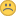# Need a type conversion mechanism to convert between strings and numbers

XMLWordPrintableJSON

#### Details

•Improvement
• Status: Closed
•Major - P3
• Resolution: Duplicate
• 2.5.3
• None
• Query
• Query 12 (04/04/16), Query 13 (04/22/16), Query 15 (06/03/16), Query 17 (07/15/16)

#### Description

Syntax

 `{\$toInt: }` `{\$toLong: }` `{\$toDouble: }` `{\$toDecimal: }` ```{\$toString: } ```

Examples

 `> db.coll.insert([` ` {_id: 0, x: "3.4"},` ` {_id: 1, x: 0.99}` `]);` `> db.coll.aggregate([{` ` \$project: {` ` intField: {\$toInt: "\$x"}` ` }` `}])` `{_id: 0, intField: 3}` `{_id: 1, intField: 0}` ` ` `// Example 2` `> db.coll.insert([` ` {_id: 0, x: {a: "b"}},` ` {_id: 1, x: 1.22},` ` {_id: 2, x: "abc"}` `]);` `> db.coll.aggregate([{` ` \$project: {` ` stringField: {\$toString: "\$x"}` ` }` `}])` `{_id: 0, stringField: "{a: 'b'}"}` `{_id: 1, stringField: "1.22"}` ```{_id: 2, stringField: "abc"} ```

Notes

• Each numeric conversion can convert from any numeric type or from a string (truncating if necessary).
• Calling \$toInt on an int is a no-op, similarly for other conversions.

Errors

• If the input is of a type or value that cannot be converted:
\$toInt, \$toLong, \$toDouble, \$toDecimal accept only int, long, double, decimal, and strings that can be interpreted as the above. \$toString accepts anything that can be turned into output that is parseable by the mongo shell.

Old Description
There are situations where I want to use a number as (part of) a string but \$concat will only take strings and not numbers. And there are other times where I have a string of a number "2012" and I want to use it as a number and there is no operator to do this.

Seems painful to fall back to map/reduce solely because someone saves a number or date as a string#### PeopleBacklog - Query Team (Inactive)Asya Kamsky